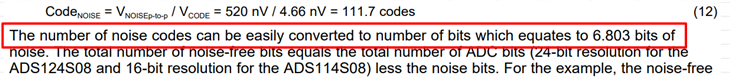If you have a related question, please click the "Ask a related question" button in the top right corner. The newly created question will be automatically linked to this question.

# ADS124S06: How to convert codes to bits?

Hi there,

We are using ADS124S06IPBS for Bridge sensor.Mentioned as noise codes are converted into bits (i.e.,) 117.7 codes into 6.803 bits.

How do we convert this? Is there any formula for this conversion.

Thanks & Regards,

Sneha.K

• Hi Sneha.K,

This is a conversion from base10 to base2.  You can use long division to determine you many times 2 divides into the decimal number.  However it is usually easier with a log conversion such as log2 (decimal number).  However many calculators will not make this conversion directly (unless you can find one online).  You can take the log10(decimal number) / log10(2).

For this example we can take log10(117.7) / log10(2) = 2.07 / 0.301 = 6.87 which is now equivalent to the number of bits.  To verify your calculation you simply convert back to decimal 2^6.87 = 117 codes.  Here we see some rounding error, but it is close enough.

Best regards,

Bob B

• Thank for your clarification Bob. It is needy one.